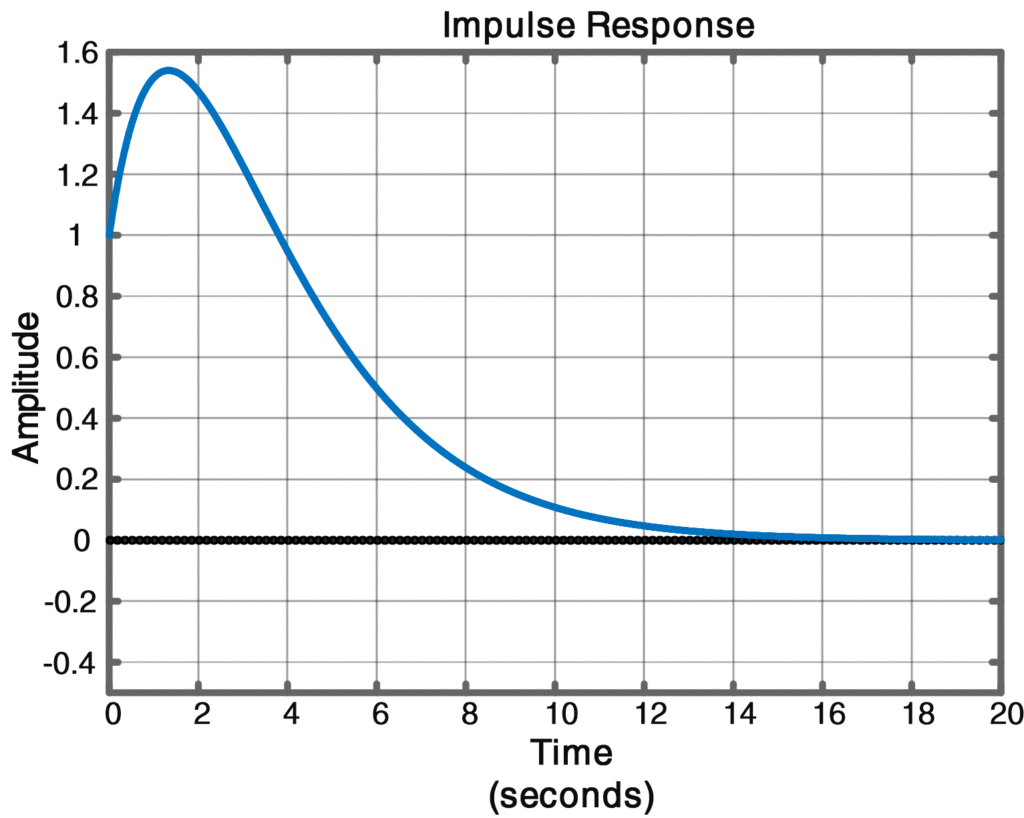Home / Control with Matlab / Impulse Response due to Repeated Poles | Matlab

# Impulse Response due to Repeated Poles | Matlab

Want create site? Find Free WordPress Themes and plugins.

## ­Time Response Due to Repeated Poles

Up to this point the discussion has been restricted to distinct poles. Either real or complex. For a repeated pole there will be more than one term in the time response, with the number of terms depending on the multiplicity of the pole. For a pole at s = p of multiplicity m = 2, we can write the Laplace transform of the response as

$\begin{matrix} G(s)=\frac{A}{{{(s-p)}^{2}}}+\frac{B}{s-p}+terms\text{ }involving\text{ }other\text{ }poles & \cdots & (1) \\\end{matrix}$

Where

\begin{align} & A={{\left. [{{(s-p)}^{2}}G(s)] \right|}_{s=p}} \\ & and \\ & B={{\left. \left\{ \frac{d}{ds}[{{(s-p)}^{2}}G(s)] \right\} \right|}_{s=p}} \\\end{align}

For t > 0, the corresponding impulse response is

$\begin{matrix} g(t)=At{{e}^{pt}}+B{{e}^{pt}}+terms\text{ }involving\text{ }other\text{ }terms & \cdots & (2) \\\end{matrix}$

Similar relationships exist for poles of multiplicity three or higher, but they are seldom needed. The residue command is able to compute the coefﬁcients for repeated poles, and for m = 2 they are listed as B followed by A as can be seen in (2).

## Impulse Response due to Repeated Poles Matlab Example

For the system whose differential equation is

$\overset{..}{\mathop{y}}\,+\overset{.}{\mathop{y}}\,+0.25y=\overset{.}{\mathop{u}}\,+2u$

Do a partial fraction expansion and write the impulse response as the sum of two individual functions of time.

Solution

The transfer function is

$\begin{matrix} G(s)=\frac{s+2}{{{s}^{2}}+s+0.25}=\frac{s+2}{{{(s+0.5)}^{2}}}=\frac{A}{{{(s+0.5)}^{2}}}+\frac{B}{s+0.5} & \cdots & (3) \\\end{matrix}$

Which has a pole at s=-0.5 of multiplicity m=2. Using the residue command results in the column vectors

 resG = polG = 1.0000 -0.5000 1.5000 -0.5000

And the scalar otherG = []. Associating the ﬁrst element of resG with A and the second element with B, we can write

$G(s)=\frac{1.0}{{{(s+0.5)}^{2}}}+\frac{1.5}{s+0.5}$

From (1) and (2), where there are no other poles, we see that for t>0 the impulse response is

$g(t)=1.0t{{e}^{-0.5t}}+1.5{{e}^{-0.5t}}$

## Matlab Code for Calculating and Plotting Impulse Response due to Repeated Poles


% Script 4: Matlab Code to Compute & Plot Impulse Response Function

clear all;close all;clc

numG = [1 2]; denG = [1 1 0.25]; % Numerator & Denumerator from text (Equation (3))

[resG,polG,otherG] = residue(numG,denG) % residues and poles computation

impulse(numG,denG)  % plotting impulse response



ResultDid you find apk for android? You can find new Free Android Games and apps.